/

### Function Description

Returns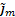, the m’th weighted percentile (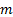here being between 0 and 1) of a series of observations series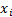given weights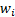(for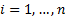).

Different commentators use different ways of identifying percentiles (i.e. quantiles) from finite sized samples. See MnPercentile and MnPercentileExc for details of how the Nematrian website calculates equally-weighted percentiles, which match the approaches used by Microsoft.

For weighted percentiles, the Nematrian website uses the following approach, which is not fully compatible with the approach it uses for unweighted percentiles:

-          First sort the series in ascending order

-          Next build up a cumulative distribution based on the weights, and assume that the percentile points for the mid-points of each bin are thein question (so, if the weight of the first in the ordered list is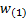then the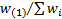’th percentile is deemed to be exactly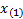, the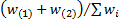’th percentile is deemed to be exactly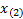, etc.)

-          Finally use linear interpolation as per the MnLinearInterpolation function to interpolate remaining percentiles.

The MnLinearInterpolation function does not extrapolate beyond the minimum and maximum values, so the 0’th and 1’th quantiles should be the minimum and maximum values of the series, and hence be consistent with what would be returned for these quantile values by the MnPercentile function. However, intermediate values with theall equal will not correspond exactly to those that would be returned by the MnPercentile function.

Contents | Prev | Next